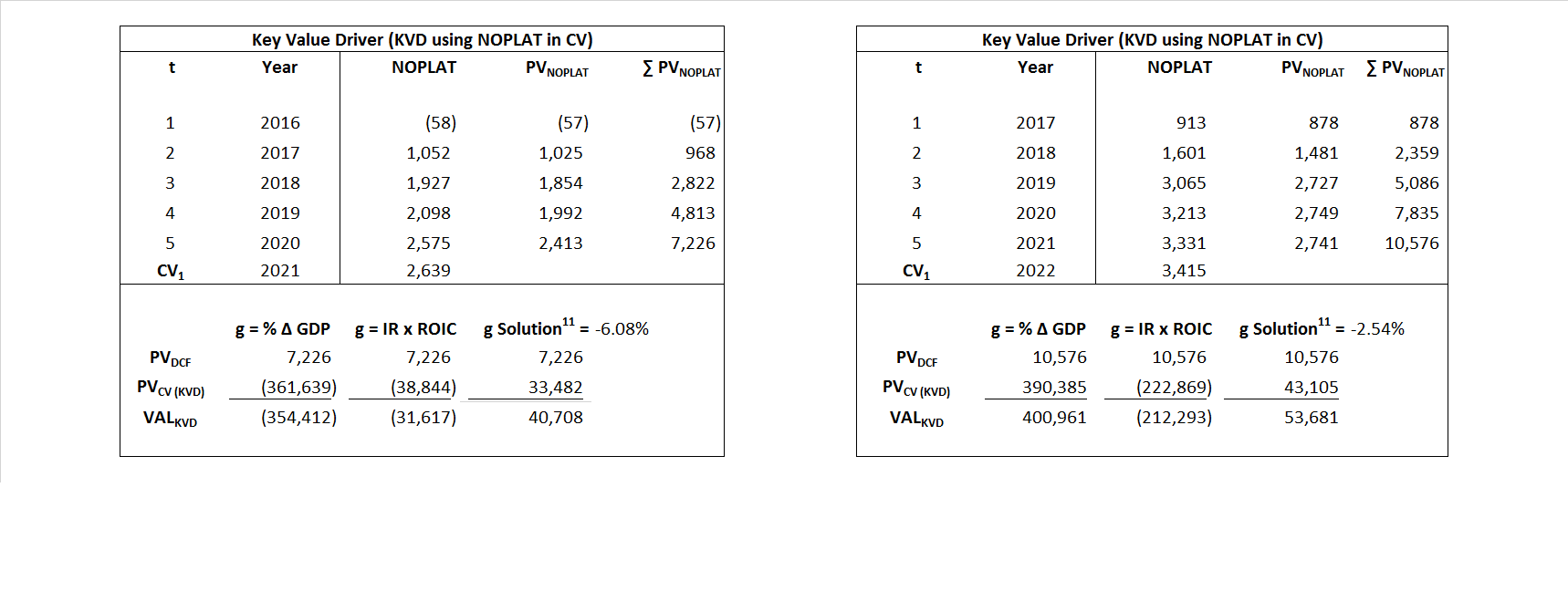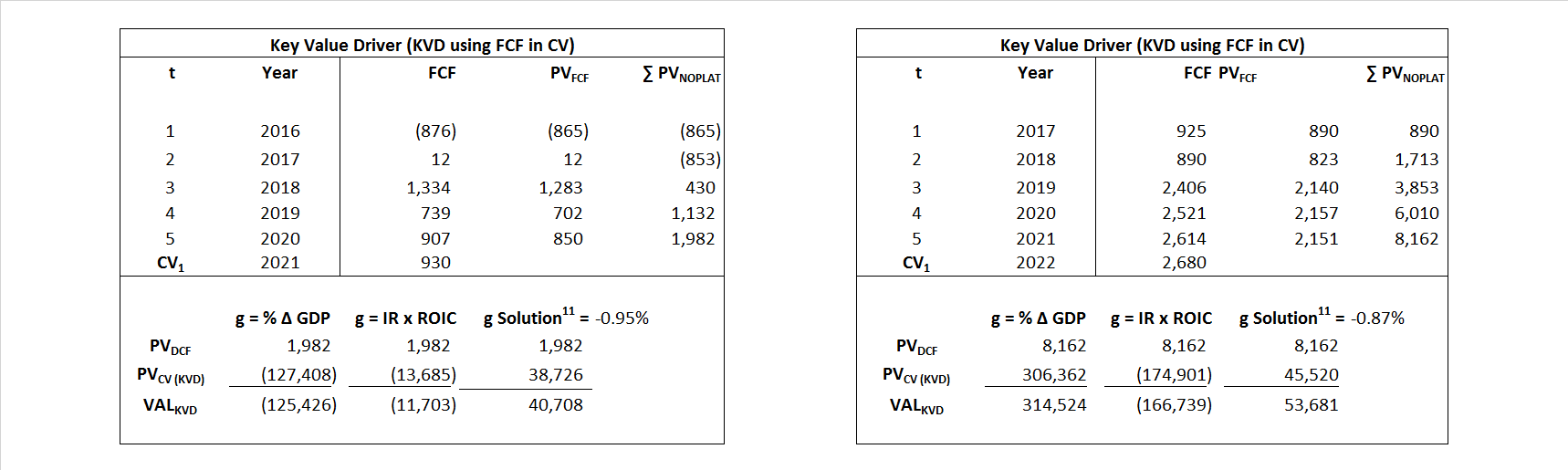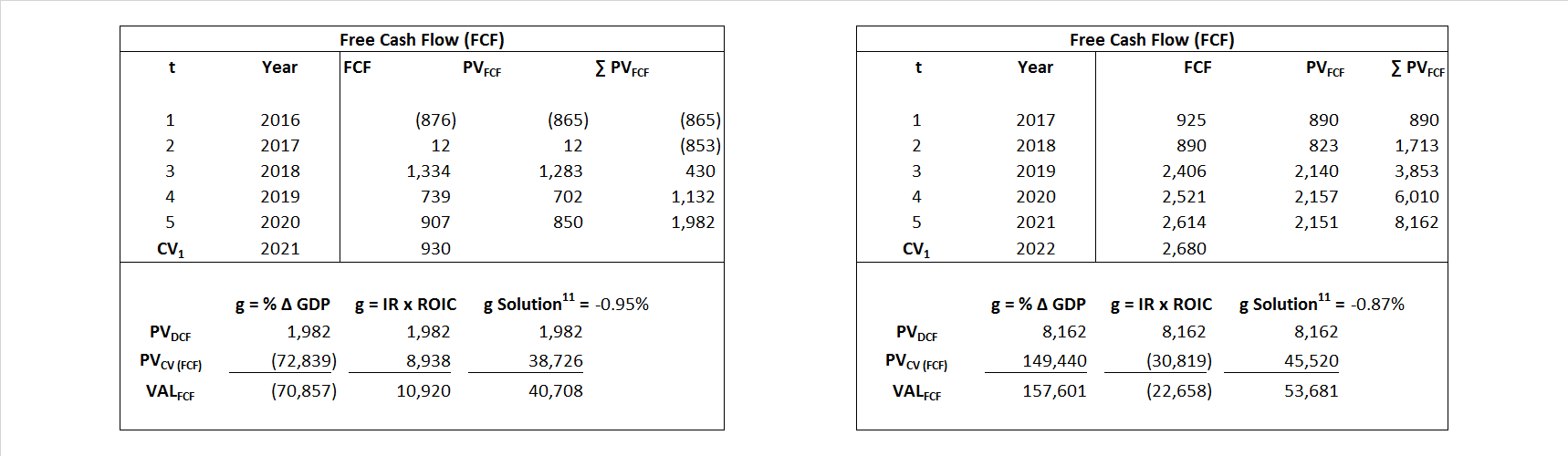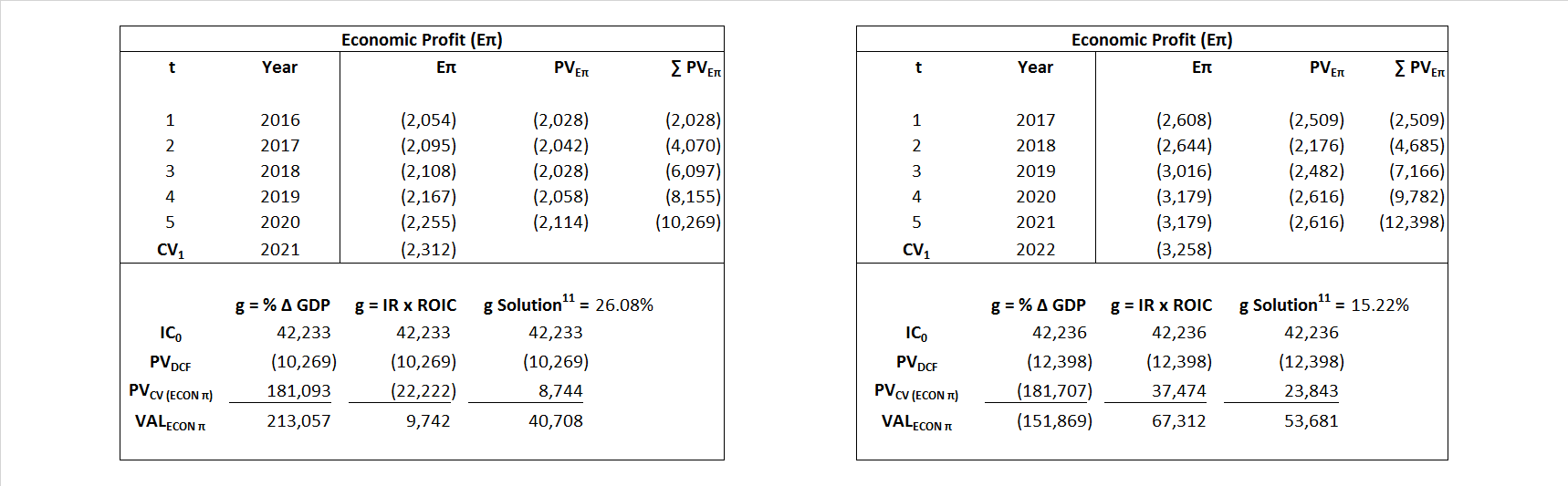## Analyst Listing

The following analysts provide coverage for the subject firm as of May 2016:

 Broker Analyst Analyst Email Tudor Pickering Holt & Co. Matthew Portillo mportillo@tphco.com Cowen & Company Charles Robertson charles.robertson@cowen.com Guggenheim Securities Subash Chandra subash.chandra@guggenheimpartners.com Stifel Nicolaus Michael Scialla mscialla@stifel.com Societe Generale John Herrlin john.herrlin@sgcib.com Deutsche Bank Research Ryan Todd ryan.todd@db.com BMO Capital Markets Phillip Jungwirth phillip.jungwirth@bmo.com Johnson Rice & Company Charles Meade cmeade@jrco.com RBC Capital Markets Scott Hanold scott.hanold@rbccm.com MUFG Securities Americas Michael McAllister michael.mcallister@mufgsecurities.com Wells Fargo Securities David Tameron david.tameron@wellsfargo.com Raymond James John Freeman john.freeman@raymondjames.com Ladenburg Thalmann & Co Michael C. Schmitz mschmitz@ladenburg.com Atlantic Equities Barry MacCarthy b.maccarthy@atlantic-equities.com IBERIA Capital Partners Eric Fox eric.fox@iberiabank.com Drexel Hamilton Robert L. Christensen Jr. rchristensen@drexelhamilton.com Alembic Global Advisors James Sullivan james.sullivan@alembicglobal.com KLR Group John Gerdes jjg@klrgroup.com Wolfe Research Paul Sankey psankey@wolferesearch.com Scotia Howard Weil Peter Kissel peter.kissel@scotiabank.com Seaport Global Securities Michael Kelly mkelly@seaportglobal.com Credit Suisse Edward Westlake edward.westlake@credit-suisse.com Capital One Securities Phillips Johnson phillips.johnston@capitalone.com

## Primary Input Data## Derived Input Data

### Equational Form

Net Operating Profit Less Adjusted Taxes NOPLAT  (1,409)  (1,006)$NOPLAT\, =\, EBIT\, x\, (1 \,-\, Avg \,\,Tax\,\, Rate\,\, on\,\, EBIT)$
Free Cash Flow FCF  (7,944)  (505)$FCF\,=NOPLAT\,+\,Non-Cash\,Expenses-\Delta NWC\,-\,NCS$
Tax Shield TS  259  234$TS\,=\,Interest\,\,Paid\,\,x\,\, Avg \,\,Tax\,\,Rate\,\, on\,\, Pre-Tax\,\, Income$
Invested Capital IC  42,233  42,236$IC\,=\,Fixed\,\,Operating\,\,Assets\,\,+\,\,Net\,\, Working\,\, Capital$
Return on Invested Capital ROIC -3.34% -2.38%$ROIC\,=\,\frac { NOPLAT }{ IC }$
Net Investment NetInv  (3,567)  4,701$NetInv\,=\,{ {IC}_{1}}-{{IC}_{0}}+Depreciation$
Investment Rate IR 253.10% -467.51%$IR\,=\,\frac {NetInv}{NOPLAT}$
Weighted Average Cost of Capital WACCMarket 1.30% 3.98%$WACC\,=\,\frac { E }{ V } { R }_{ E }\,+\,\frac { P }{ V } { R }_{ P }\,+\,\frac { D }{ V } { R }_{ D }\left( 1- Avg\,\, Tax\,\,Rate\,\,on\,\,Pre-Tax\,\,Income \right)$
WACCBook  8.80% 9.81%
Enterprise value EVMarket   40,708  53,681$EV\,=\,Market\,\,Cap\,\,Equity\,+\,\,Long\,\,Term\,\,Debt\,-\,Cash$
EVBook  39,472  50,532
EV/EBIT Multiple$\frac{EV_{Market}}{EBIT}$  (24.55)  (45.38)$EV/EBIT\,=\,\frac { EV}{ EBIT}$
Long-Run Growth g = IR x ROIC
-8.45%    11.13% Long-run growth rates of the income variable  are used in the Continuing Value portion of the valuation models.
g = %$\Delta$ GDP     2.50%    2.50%

## Valuation Model Outcomes

The outcomes presented in this study are the result of original input data, derived data, and synthesized inputs and, depending on the equational form of any particular valuation model, may result in irrelevant or implausible results.  For example, in the event WACC < g, the value of this term, often found in the denominator of an equation’s continuation value term, will be expressly negative and may result in a negative overall valuation for the firm.  In the event of a WACC < g relation, the model form as applied to the subject firm offers an irrelevant outcome.

### Equational form

Key Value Driver (NOPLAT) KVD (NOPLAT)${ Value }_{ DCF/KVD }=\sum { \frac { NOPLAT_{ t } }{ { \left( 1+WACC \right) }^{ t } } +\frac { \frac { { NOPLAT }_{ 1 }\left( 1-\frac { g }{ ROIC } \right) }{ WACC-g } }{ { \left( 1+WACC \right) }^{ t } } }$Key Value Driver (FCF) KVD (FCF)${ Value }_{ DCF/KVD }=\sum { \frac { FCF_{ t } }{ { \left( 1+WACC \right) }^{ t } } +\frac { \frac { { NOPLAT }_{ 1 }\left( 1-\frac { g }{ ROIC } \right) }{ WACC-g } }{ { \left( 1+WACC \right) }^{ t } } }$Free Cash Flow FCF${ Value }_{ DCF/FCF }=\sum { \frac { FCF_{ t } }{ { \left( 1+WACC \right) }^{ t } } +\frac { \frac { { FCF }_{ 1 }}{ WACC-g } }{ { \left( 1+WACC \right) }^{ t } } }$Economic Profit ECON π${ Value }_{ { ECON\pi } }= I{ C }_{ 0 }+\sum { \frac { { IC }_{ t-1 }(ROI{ C }_{t}-WAC{C}_{t}) }{ { \left( 1+WACC \right) }^{ t } }+ \frac {\frac { I{C}_{0}\ x\ (ROI{C}_{1}\ -\ WAC{C}_{1}) }{ WACC-g } }{ { \left( 1+WACC \right) }^{ t } } }$Adjusted Present Value APV${ Value }_{ APV }=\sum { \frac { FCF_{ t } }{ { \left( 1+{ k }_{ u } \right) }^{ t } } +\frac { \frac { { FCF }_{ 1 }}{ { k }_{ u }-g } }{ { \left( 1+{ k }_{ u } \right) }^{ t } } } +\sum { \frac { { TS }_{ t } }{ { \left( 1+{ k }_{ tax } \right) }^{ t } } +\frac { \frac { { TS }_{ 1 }}{ { k }_{ tax }-g } }{ { \left( 1+{ k }_{ tax } \right) }^{ t } } }$Forward Market Multiple FMM${ Value }_{ DCF/FMM}=\sum { \frac { FCF_{ t } }{ { \left( 1+WACC \right) }^{ t } } +\frac { { EBIT }_{ 1 }\,{x}\,{FMM}}{ { \left( 1+WACC \right) }^{ t } } }{\,\,\,; \,\,FMM\,=\,\frac{{EV}_{t=0}}{{EBIT}_{t=0}}}$